Courses

# NCERT Solutions (Part- 2)- Introduction to Graphs Class 8 Notes | EduRev

## Class 8 Mathematics by VP Classes

Created by: Full Circle

## Class 8 : NCERT Solutions (Part- 2)- Introduction to Graphs Class 8 Notes | EduRev

The document NCERT Solutions (Part- 2)- Introduction to Graphs Class 8 Notes | EduRev is a part of the Class 8 Course Class 8 Mathematics by VP Classes.
All you need of Class 8 at this link: Class 8

Exercise 15.2

Question 1. Plot the following points on a graph sheet. Verify if they lie on a line:
(a) A(4, 0), B(4, 2), C(4, 6), D(4, 2.5)
(b) P(1, 1), Q(2, 2), R(3, 3), S(4, 4)
(c) K(2, 3), L(5, 3), M(5, 5), N(2, 5)

Solution:
In each case we draw the x-axis and the y-axis and plot the given point.
(a) on plotting the points A(4, 0), B(4, 2), C(4, 6) and D(4, 2.5) and then joining them we find that they all lie on the same line.
(b) On plotting the points P(1, 1), Q(2, 2), R(3, 3) and S(4, 4) and then joining them we find that they all lie on the same line.
(c) Potting the points k(2, 3) L(5, 3), M(5, 5) and N(2, 5) and joining them we find that all of them do not lie on the same line.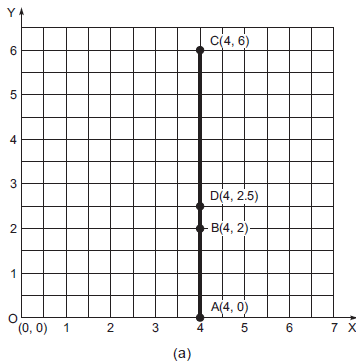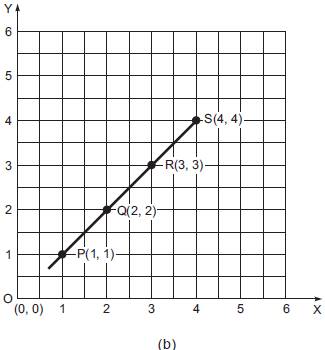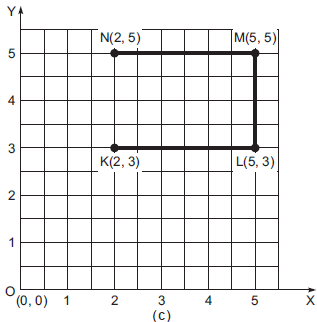Question 2. Draw the line passing through (2, 3) and (3, 2). Find the coordinates of the points at which this line meets the x-axis and y-axis.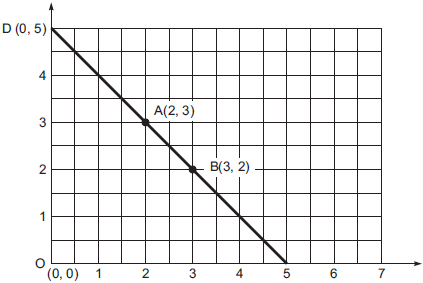Solution: On plotting the points A(2, 3) and B(3, 2) and joining them, draw a line. On extending the line meets the x-axis at c(5, 0) and the y-axis at D(0, 5).

Question 3. Write the coordinates of the vertices of each of these adjoining Figures.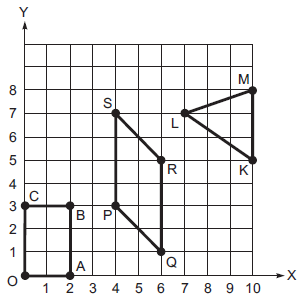Solution:
(i) The co-ordinates of:
O are (0, 0)
A are (2, 0)
B are (2, 3)
C are (0, 3)

(ii) The co-ordinates of:
P are (4, 3)
Q are (6, 1)
R are (6, 5)
S are (4, 7)

(iii) The co-ordinates of:
K are (10, 5)
L are (7, 7)
M are (10, 8)

Question 4. State whether true or false. Correct that are false.
(i) A point whose x coordinate is zero and y-coordinate is non-zero will lie on the y-axis.
(ii) A point whose y coordinate is zero and x-coordinate is 5 will lie on y-axis.
(iii) The coordinates of the origin are (0, 0).
Solution: (i) True (ii) False (iii) True

Question: The number of litres of petrol you buy to fill a car’s petrol tank will decide the amount you have to pay. Which is the independent variable here? Think about it.
Solution: Since, quantity of petrol is a need whereas the amount of money is linked with the quantity of petrol.
∴ Petrol is the independent variable.

Question: The following table gives the quantity of petrol and its cost.

 No. of litres of petrol 10 15 20 25 Cost of petrol in Rs 500 750 1000 1250

Plot a graph to show the data.
Use the graph to find how much petrol can be purchased for Rs 800.
Solution: We draw axes and take a suitable scale on both the axes. To draw the graph, we take the following steps:
I. Mark the number litres of petrol along the horizontal axis.
II. Mark the cost of petrol (in rupees) along the y-axis.
III. Now, we plot the given points, i.e. (10, 500); (15, 750); (20, 1000); (25, 1250)
IV. Join the points.
We find that the graph is a straight line.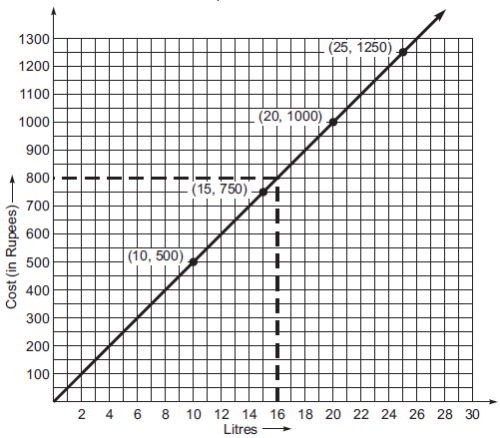Yes, we can find the quantity of petrol to be got in Rs 800, for this take a point on the y-axis (0,800). Through A draw BC parallel to x-axis to meet the graph at B. Now from B, draw BC Perpendicular x-axis.
As C corresponds to (16, 0).
Thus, 16 litre petrol can be bought for Rs 800.

Exercise 15.3
Question 1. Draw the graphs for the following tables of values, with suitable scales on the axes.
(a) Cost of apples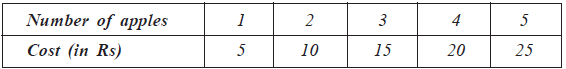(b) Distance travelled by a car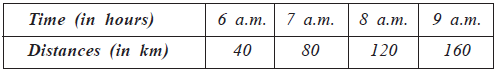(i) How much distance did the car cover during the period 7.30 a.m. to 8 a.m.?
(ii) What was the time when the car had covered a distance of 100 km since it’s start?

(c) Interest on deposits for a year.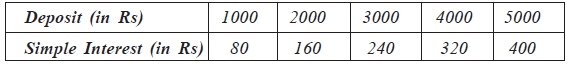(i) Does the graph pass through the origin?
(ii) Use the graph to find the interest on Rs 2500 for a year.
(iii) To get an interest of Rs 280 per year, how much money should be deposited?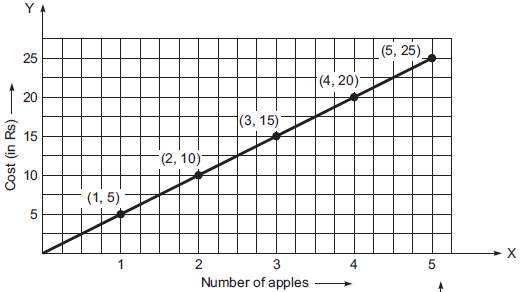Solution: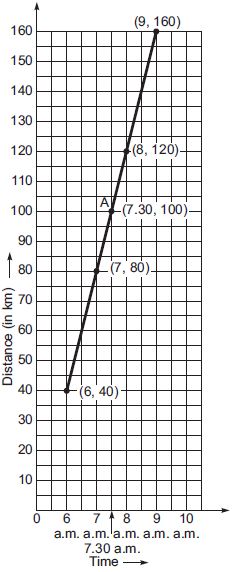(a) I. Draw x-axis and y-axis mutually perpendicular to each other.
II. Take a suitable scale.
III. Take the number of apples along the x-axis and mark the cost (in Rs) along the y-axis.
IV. Plot the points (1, 5), (2, 10), (3, 15), (4, 20) and (5, 25)
V. Joint the points.
We obtain the graph a straight line.

(b) I. Draw the axis.
II. Choose suitable scale along x-axis and along y-axis.
III. Mark time (in hours) along x-axis and distance (in km) along y-axis
IV. Plot the points (6, 40) (7, 80), (8, 120) and (9, 160).
V. By joining the points we get the required graph.
(i) In the graph, draw a perpendicular at the point indicating 7:30 a.m. on the x-axis such that it meets the graph at A. From A draw a line parallel to x-axis to meet y-axis at 100 km.
∴ Distance travelled between 7:30 a.m. and 8:00 a.m.
= 120 km – 100 km
= 20 km
(ii) 7:30 a.m.

(c) I. Draw axes.
II. Take appropriate scale on x-axis and y-axis.
III. Mark the deposits along the x-axis.
IV. Mark the interest along the y-axis.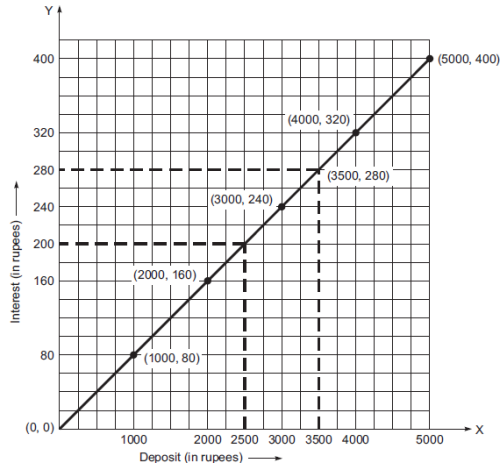V. Plot the point (1000, 80) (2000, 160), (3000, 240), (4000, 320) and (5000, 400).
VI. Join the points and get the graph. Now from the graph, we have:
(i) Yes, it passes through the origin
(ii) From the graph, the interest on Rs 2500 is Rs 200.
(iii) From the graph an interest of Rs 280 can be got by depositing Rs 3500.

Question 2. Draw a graph for the following.
(i)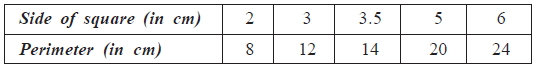Is it a linear graph?
(ii)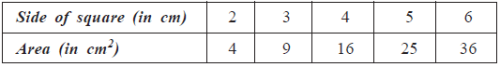Is it a linear graph?

Solution: (i) Taking the side of the square along the x-axis and the perimeter along the y-axis and plotting the points (2, 8), (3, 12), (3.5, 14), (5, 20) and (6, 24), we get the required graph as a straight line.
∴ This graph is a linear graph.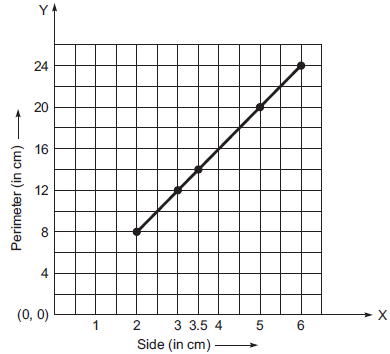(ii) Taking the side of the square along the x-axis and area (in cm2) along the y-axis, we can draw the required graph by plotting the points (2, 4), (3, 9), (4, 16), (5, 25) and
(6, 36) as shown in the following figure.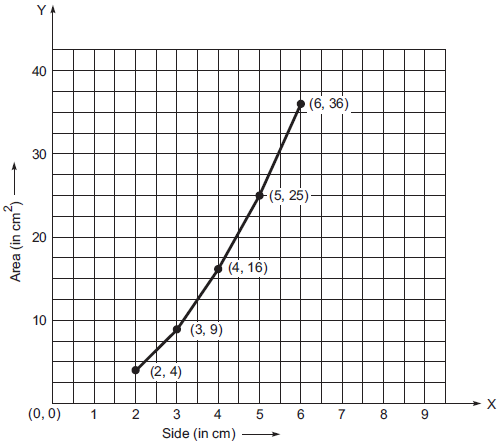∵ The graph in not a straight line.
∴ It is not a linear graph.

93 docs|16 tests

,

,

,

,

,

,

,

,

,

,

,

,

,

,

,

,

,

,

,

,

,

;